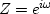Next: Causality and the unit Up: INSTABILITY Previous: The meaning of divergence

## Boundedness

Given different numerical values for Z, we can ask whether X(Z) is finite or infinite. Numerical values of Z of particular interest are Z = +1, Z = -1, and all those complex values of Z which are unit magnitude, say |Z| = 1 or,whereis the real Fourier transform variable. Whenis the variable, the Z-transform is a Fourier sum.

We can restrict our attention to those signals ut that have a finite amount of energy by demanding that U(Z) be finite for all values of Z on the unit circle |Z| = 1. Filter functions always have finite energy.Next: Causality and the unit Up: INSTABILITY Previous: The meaning of divergence
Stanford Exploration Project
10/21/1998